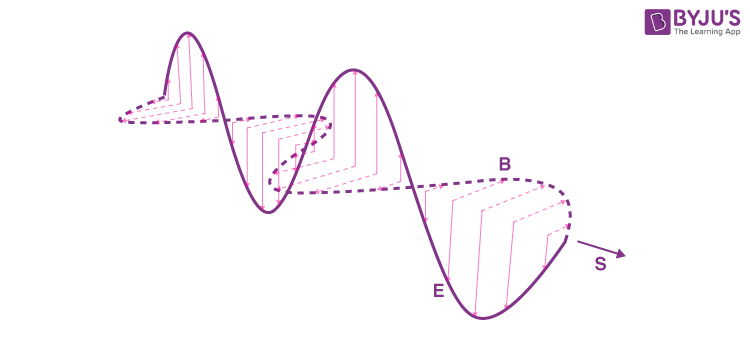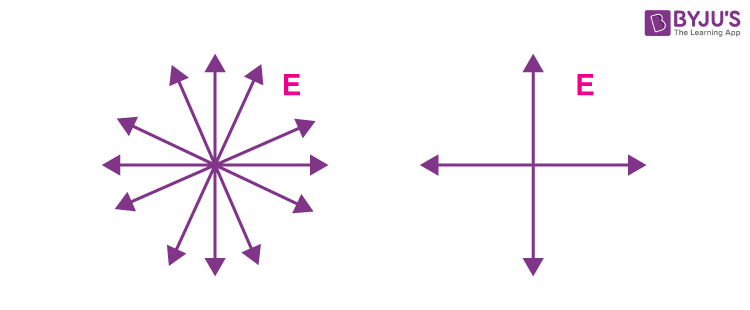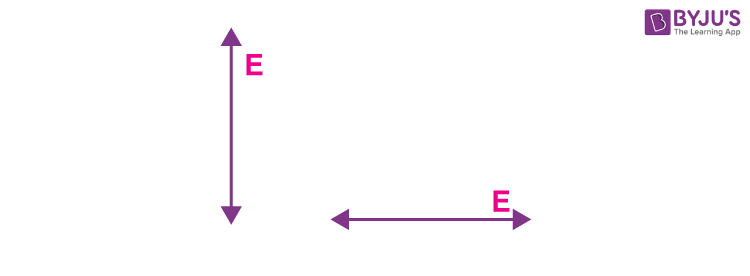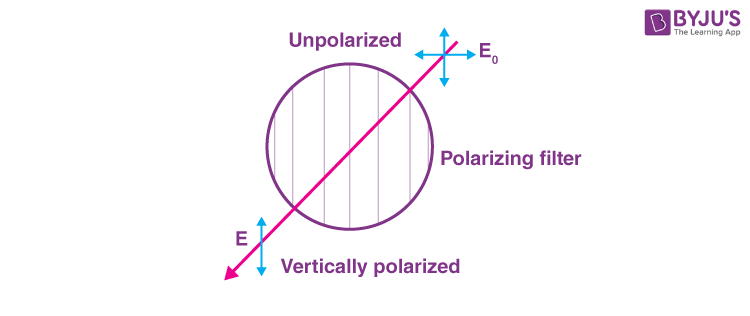# Polaroid Films - Light Filtering via Polarization of Light

## What is Polaroid?

Light can be thought to be a sinusoidal oscillation of the electromagnetic fields. The electric and magnetic fields are both perpendicular to the direction of propagation and are themselves perpendicular to each other. The pointing vector vector S gives the direction of propagation as perpendicular to the two fields as:

$$\begin{array}{l}\overrightarrow{S} = \frac{1}{\mu}\overrightarrow{E}~\times~\overrightarrow{B}\end{array}$$The electric field can be said to be oscillating along a plane perpendicular to vector S. However, there are infinitely many such planes, and light that contains fields randomly distributed along all these planes are said to be unpolarized as shown. Since the electric fields can be vectorially resolved along with any perpendicular directions, the representation of unpolarized light can be shown as on the right.Plane polarized light, on the other hand, contains light with the electric field (and hence the magnetic field) along only one plane as shown below:## What is the Plane of Polarization?

A polarizing filter produces polarized light from unpolarized light by selectively allowing electric fields (and thus magnetic fields) along a single plane known as the plane of polarization.

### What is Polarizing Direction?

The direction of the electric field upon polarization is known as the polarizing direction.

#### Polarizing Sheets:

Polarizing sheets or polarizing filters are commercially known as polaroids. These sheets contain long-chain molecules that are aligned to allow electric field oscillations along only one direction. Early polarizing sheets comprised of aligned crystals embedded in plastics. Today, specially treated organic molecules in films are used more commonly as polarizing sheets. The direction along which the filter polarizes light is its polarizing direction.

The intensity of fully unpolarized light upon passing through a polarizing sheet is half the original intensity ie,

$$\begin{array}{l}I = \frac{1}{2}~I_0\end{array}$$If a filter encounters plane-polarized light at an angle θ to its polarizing direction, only the component of the electric field along the polarizing direction is passed along i.e.,

$$\begin{array}{l}E = E_0~Cosθ\end{array}$$
The intensity is proportional to the square of the electric field. Thus,

$$\begin{array}{l}I~∝~E^2 = E_0^2~Cos^2~θ\end{array}$$

$$\begin{array}{l}I = I_0~Cos^2~θ\end{array}$$

This ability of these filters to reduce the intensity of light is the primary use of Polaroid.

### Uses

• Polaroid films are commonly used to reduce the glare of sunlight in bright settings. Since light reflected from water is partially polarized, these filters are used when photographing water bodies and when photographing objects underwater.Note the increased contrast on the right.

• Polaroid is used on spectacles to reduce glare. They are also used in night driving goggles as they can filter out the partially polarized light reflected off windshields.
• Plane polarized light is commonly used in chemical analysis to determine the chirality or handedness of molecules. When such light passes through a solution of chiral molecules, they rotate the plane of polarization by a particular angle. By determining the angle of rotation in a solution, it is possible to determine the purity of products obtained from a chemical reaction.
• Plane polarized light is used to produce circularly polarized light. In this case, the electric vector rotates as it propagates. By introducing a phase shift using a birefringent material, it is possible to polarize light circularly.

Stay tuned with BYJU’S to learn more about electromagnetic waves, the polarization of light, and much more.

Test your knowledge on Polaroid films